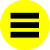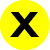# tera-

In units of measurement, a factor of 1012, a million million.

Greek teras, monster.

This is one of the standard SI (Système International) multiples (see the entryNumber words). It occurs in terms such as terahertz, a frequency of a million million cycles per second, and terawatt, a million million watts. In computing, it strictly has a sense of 240, but in terms such as terabyte it often means a million million bytes. The unit of computing speed, the teraflop, is always equal to one million million floating-point operations per second.